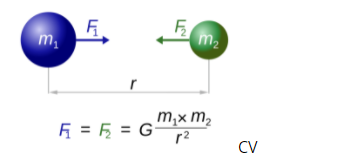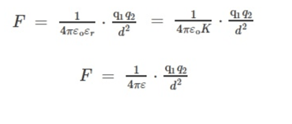Monday, October 2, 2023
HomeEducationEverything You Need to Know About Non-Contact Forces With Examples

# Everything You Need to Know About Non-Contact Forces With Examples

Force is the interaction between objects. It has both -magnitude and direction. When two objects interact, each object applies a force to the other. Any push or pull leads to a change in an object’s state of motion, direction, or shape. The unit in which force is measured in Newton (N), named after Sir Isaac Newton.

Force can be classified into two  different  types based on how  it  is  exerted:

1. Contact force
2. Non-contact force

Any force that requires contact between two bodies to occur is contact-force. An object can exert it to another only by physical contact between the two. Applied force, normal force, muscular force, and frictional force are some types of contact forces.

Contact force occurs when we push a car uphill or kick a football across a playground. Moreover, it is essential to know that the origin of all contact forces (such as frictional force) can be traced back to non-contact forces.

### What is a non-contact force?

Non-contact force is applied to an object by another body that is not in direct contact with it. Either attraction or repulsion between two objects that are not in direct contact with each other leads to non-contact force. These forces are invisible by nature, and they can act without needing any physical effort. There is always a distance between the two interacting objects. There are few non-contact forces as compared to contact forces. A field is associated with the non-contact force, unlike the contact force where no field is associated.

### Examples of Non-contact Forces

#### Gravitational Force

The gravitational force of gravity is the universal force of attraction acting between all matter. It is a natural phenomenon because all bodies with mass or energy, including planets, stars, and galaxies, are attracted to each other. It is one of the most familiar types of non-contact forces. Gravitational force is weaker than other non-contact forces; however, it has the longest (infinite) range.

Raindrops falling on the earth is an example of a gravitational force. The two objects, i.e. raindrops and earth, are not physically in contact; hence it is a non-contact force.

Another example is leaves and fruits falling downwards on the ground due to the gravitational pull. One of the simplest examples is that we land back on the ground after jumping upwards.

The formula for the gravitational force between two objects is:Where F is the force,

G is the gravitational constant,

m1 and m2 are the masses of the two objects, and

r is the distance between the centres of the masses.

#### Magnetic Force

Magnetic force is the attraction or repulsion exerted between the two poles of a magnet and electrically charged moving particles. It is a result of electromagnetic forces, which is one of the four fundamental forces of nature.

The magnetic force that occurs between two magnets can either be a force of attraction or that of repulsion, depending upon which of the poles of the two given magnets face each other. There exists a magnetic force of repulsion between two similar poles of two magnets. And there exists a magnetic force of attraction between two unlike poles of two magnets.

An example of magnetic force can be seen when a magnet attracts metal objects like iron and iron filings towards itself. Since this interaction between two objects occurs from a distance, the magnetic force is known as a type of non-contact force.

Another example is the refrigerator door, which remains closed due to the magnetic force of attraction between the magnetic strips on the door.

#### Electrostatic Force

The force that is exerted by an electrically charged object is known as the electrostatic force. The electric charges can be positive or negative (or attractive or repulsive) in nature. They can be produced by friction, which arises when one object is rubbed against another object.

The electrostatic force is known as a non-contact force because these forces come into play even when the objects are not in contact with each other. It is also known as Coulomb’s force and is much stronger than the gravitational force. However, it is weaker than the nuclear force.

An example of the electrostatic force is when we comb our hair and place pieces of paper near the comb. The pieces of paper are drawn to the plastic comb because it picks up charges from the hair.

Another example is when nylon clothes are rubbed against the wearer’s skin; static electricity is formed, raising the hair on the skin. It also causes the chattering sound that occurs while removing the nylon cloth.

The formula for calculating electrostatic force using Coulomb’s law is:Where F is the force,

k is the coulomb’s constant,

q1 and q2 are the magnitudes of the two electric charges, and

r  is the distance between the centres of two charges.

#### Nuclear Force

The nuclear force is another type of non-contact force that acts between two or more nucleons. This force is what holds the nucleus together, as it binds the protons and neutrons. It can also exist between protons and protons, or neutrons and neutrons.

The strong nuclear force is also known as strong interaction. This force plays an essential role in energy storage that is prevalent in nuclear power and nuclear weapons. It is also one of the four fundamental forces of nature, and it is the strongest of them.

It also has the shortest range, which means that the particles should be extremely close to feel their effects. The nuclear force is attractive but can also be effectively repulsive in certain conditions. This force is independent of charge, unlike the electrostatic force.

A prime example of nuclear force in action can be seen in the destructive power of nuclear weapons. Another example is the generation of heat for generating electricity that can be done with the help of nuclear power plants.

#### Conclusion

Thus, non-contact forces are very important. They form a significant part of our everyday life, and several examples from daily life can be observed. Learning about this is critical to know more about the usage of science in the real world.sachin
He is a Blogger, Tech Geek, SEO Expert, and Designer. Loves to buy books online, read and write about Technology, Gadgets and Gaming. you can connect with him on Facebook | Linkedin | mail: srupnar85@gmail.com